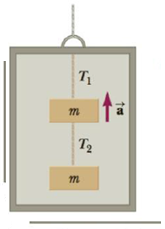Chapter 4, Problem 56P

Chapter
Section
Textbook Problem

Two blocks each of mass m are fastened to the top of an elevator as in Figure P4.56. The elevator has an upward acceleration a. The strings have negligible mass, (a) Find the tensions T1 and T2 in the upper and lower strings in terms of m, a, and g. (b) Compare the two tensions and determine which string would break first if a is made sufficiently large. (c) What are the tensions if the cable supporting the elevator breaks?Figure P4.56

(a)

To determine
The tension on the upper and lower strings.

Explanation

Given Info: Masses attached are m. Acceleration of the elevator is a.

The free body diagram is given below.

From the diagram,

T1T2mg=ma (I)

T2mg=ma (II)

• m is the mass of the block.
• g is the acceleration due to gravity.
• a is the acceleration.

On Re-arranging Equation (II),

T2=m(a+g)

On Re-arranging Equation (I),

T1=m(a+g)+T2

Substitute m(a+g) for T2 in the above expression to get T1

(b)

To determine
The ratio of the T1 and T2 .

(c)

To determine
The tensions when the string breaks.

Still sussing out bartleby?

Check out a sample textbook solution.

See a sample solution

The Solution to Your Study Problems

Bartleby provides explanations to thousands of textbook problems written by our experts, many with advanced degrees!

Get Started

Lysine does not cure herpes infections. T F

Nutrition: Concepts and Controversies - Standalone book (MindTap Course List)

True or false? Ribosomes are only found in eukaryotes.

Biology: The Unity and Diversity of Life (MindTap Course List)

13-27 Define autoxidation.

Introduction to General, Organic and Biochemistry